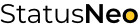WE ARE EXPERTS IN TECHNOLOGY

## Let’s Work Together# StatusNeo## Excel Automation with Python’s Openpyxl: A Comprehensive Guide

Are you tired of manually processing large amounts of data in Excel? Do you want to automate your tasks and save time? Look no further! In this blog post, we’ll explore how to read and write Excel files using the `openpyxl` module in Python, along with some coding examples.

`openpyxl` is a popular Python library that provides easy-to-use methods for working with Excel files. Whether you need to read data from an existing Excel file or create a new Excel file and write data into it, `openpyxl` has got you covered. So let’s dive in!

## Installing `openpyxl`

Before we start, let’s make sure you have `openpyxl` installed. You can install it using `pip`, the Python package manager, by running the following command in your terminal or command prompt:

``pip install openpyxl``

Let’s start with reading data from an existing Excel file. Here’s an example of how you can do that using `openpyxl`:

``````import openpyxl

# Get the active sheet
sheet = workbook.active

# Access data from cells
cell_value = sheet['A1'].value
print("Cell A1 value:", cell_value)

# Access data from rows and columns
for row in sheet.iter_rows(min_row=2, max_row=5, min_col=1, max_col=3, values_only=True):
print(row)``````

In this example, we first loaded the Excel workbook using `openpyxl.load_workbook()`, passing the file name as an argument. Then, we accessed the active sheet using the `active` property of the workbook object. We can access the data in individual cells by specifying the cell’s coordinates in square brackets (e.g., `sheet['A1'].value`).

We can also access data from multiple cells using the `iter_rows()` method, which allows us to iterate through a range of rows and columns in the sheet. In this example, we iterated through rows 2 to 5 and columns 1 to 3 and accessed the values from those cells using the `values_only` parameter set to `True`.

## Writing Excel Files

Next, let’s learn how to create a new Excel file and write data into it using `openpyxl`. Here’s an example:

``````import openpyxl

# Create a new Excel workbook
workbook = openpyxl.Workbook()

# Get the active sheet
sheet = workbook.active

# Write data to cells
sheet['A1'] = 'Name'
sheet['B1'] = 'Age'
sheet['C1'] = 'City'

data = [
('Alice', 25, 'New York'),
('Bob', 30, 'Los Angeles'),
('Charlie', 35, 'Chicago'),
('David', 40, 'San Francisco')
]

for row in data:
sheet.append(row)

# Save the workbook
workbook.save('example_new.xlsx')``````

In this example, we first created a new Excel workbook using `openpyxl.Workbook()`. Then, we accessed the active sheet as before. We can write data to cells by assigning values to the cell’s coordinates (e.g., `sheet['A1'] = 'Name'`). We can also use the `append()` method to add data as rows to the sheet.

Finally, we saved the workbook using the `save()` method, specifying the file name to save the new Excel file with the written data.

## Modifying Excel Files

`openpyxl` also allows you to modify existing excel files by updating the values in cells, adding or deleting rows and columns, and formatting cells. Here’s an example:

``````import openpyxl
from openpyxl.styles import Font

# Get the active sheet
sheet = workbook.active

# Update cell values
sheet['B2'] = '26'  # Update cell B2 with a new value

new_row = ('Eve', 28, 'Seattle')
sheet.append(new_row)  # Append the new row to the end of the sheet

# Delete a row
sheet.delete_rows(3, 1)  # Delete row 3

# Format cells
font = Font(bold=True)
sheet['A1'].font = font  # Set cell A1 to bold

# Save the modified workbook
workbook.save('example_modified.xlsx')``````

In this example, we first loaded the existing Excel workbook as before. Then, we accessed the active sheet and made updates to cell values using the cell’s coordinates (e.g., `sheet['B2'] = '26'`). We also appended a new row to the sheet using the `append()` method with a tuple of values for the new row.

Next, we deleted a row using the `delete_rows()` method, specifying the starting row and the number of rows to delete. We also applied formatting to a cell using the `Font` class from the `openpyxl.styles` module. In this case, we set the font of cell A1 to bold.

Finally, we saved the modified workbook using the `save()` method, which created a new Excel file with the updates.

And that’s it! With `openpyxl`, you can easily read, write, and modify Excel files in Python, enabling you to automate your data processing tasks and save time.

## Summary

Here’s a summary of the basic steps for reading and writing Excel files using `openpyxl`:

1. Install `openpyxl` using `pip` if not already installed.
2. Load an existing Excel file using `openpyxl.load_workbook()` and access the active sheet.
3. Read data from cells using cell coordinates or iterate through rows and columns using `iter_rows()`.
4. Write data to cells using cell coordinates or append new rows using the `append()` method.
5. Modify Excel files by updating cell values, adding or deleting rows and columns, and formatting cells.
6. Save the workbook using the `save()` method to create a new Excel file with the changes.

With these steps and some basic Python programming knowledge, you can easily automate your Excel-related tasks using `openpyxl` in Python. Happy coding!